## Is there anything which establishes a mathematical correlation between two different impulse responses?Started by 6 years ago9 replieslatest reply 6 years ago112 views

Hello,

Apologies for the continued questions on convolution reverb, but I was wondering if someone could answer the following 2 questions (as I've hit a bit of a stumbling block in my convolution reverb research):

1. Is there some mathematical function which establishes a correlation between two different impulse responses acting on a shared signal (if so, what is the name of that function)?

2. Does this correlation between two impulse responses extend throughout the entire shared signal?

To clarify, let's say two people (Alice and Bob) each have their own impulse response.

Alice's IR: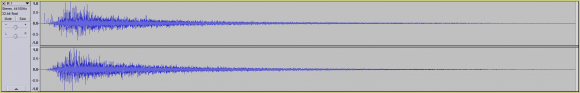Bob's IR: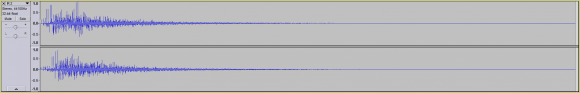Let's also say that the shared signal is the following: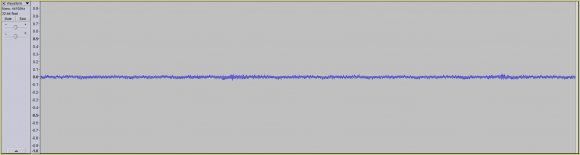When Alice convolves the shared signal with her IR, it results in the following convolved signal:Whereas when Bob convolves the shared signal with his IR, it results in the following convolved signal:What I'm trying to do is find some way of establishing a correlation between Alice and Bob's convolved signals.

Hypothetically, something like the integral of Alice's convolved signal is XXX times larger than the integral of Bob's convolved signal.

However, I need this mathematical correlation to hold throughout the entirety of the convolved signal.

In other words, if instead of having the entire convolved signal, we instead only had the 1st half of Alice and Bob's convolved signals: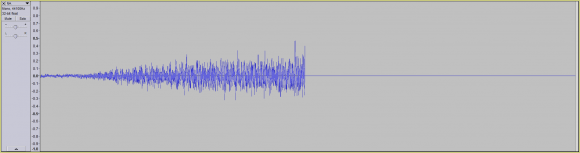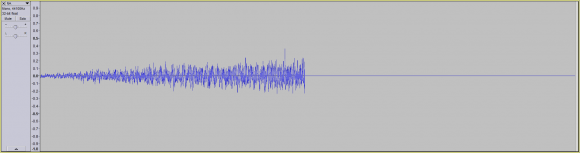...I'd need this mathematical correlation / value to still hold.

Or conversely, if we only had the last half of Alice and Bob's convolved signal:...the correlation would still need to hold, namely that the integral of Alice's convolved signal is still XXX times larger than Bob's.

Keep in mind that this doesn't need to be an integral (I was using an integral as an example), but it would need to be some mathematical way of establishing a correlation between two different IR's.

And most importantly, the correlation would need to stand if you had the entirety of the two convolved signals, or only a piece of both signals (ex. the first half of both or the last half of both).

Any help would be greatly appreciated.

Thank you,
Nelson

[ - ]Well I will use the convolution theorem to derive the correlation then further use a sort of Boxcar window to define the truncation. You will end up by having something like this F{S_a}F{S_b}=F{S_oa}F{S_ob}^-1, where F is the Fourier transform. etc (S_a : input response Alice, S_oa: output signal Alice)

[ - ]Hello Ruth,

Thank you very much for the information.

Regarding F{S_a}F{S_b}=F{S_oa}F{S_ob}^-1, will this equate to the same value whether we're using Alice and Bob's entire convolved signal:...or a partially convolved signal (ex. the 1st half of Alice and Bob's convolved signal):...or the 2nd half of Alice and Bob's convolved signal: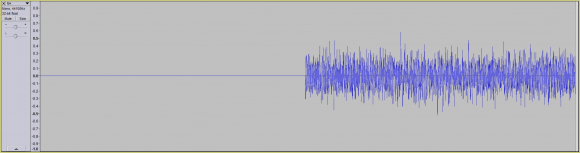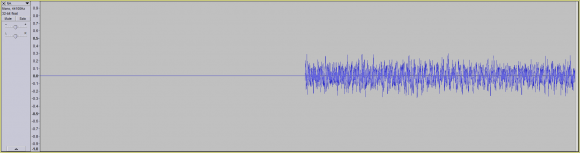If so, thank you very much for all your help.

Nelson

[ - ]It will work in theory (the signal is extended infinitely) but in practical situations, you need now to introduce a sort of box window and integrate over a define interval then it will work. NB, I have given my Honour student to test this and come back to me. We will let you know.

[ - ]Hello Ruth,

Nelson

[ - ]I can't answer you question, but I did want point something out.  If you are convolving two finite signals, the result should contain both a beginning and an end.  Given the shape of your impulse responses, I would expect the output to have a fast attack and a decay with a long tail.  The most likely causes for such an issue would be that the convolution is not being performed properly, or there is not enough zero padding on the input and/or impulse responses.  I'm guessing it's the latter.

To the point of your question, could you elaborate on what you mean by correlate

[ - ]Hello Dszabo,

Regarding my use of convolution, you are correct. The decay of the convolved signal is being truncated (I'll use examples with the full signal from now on to avoid any further confusion).

As for an elaboration of what I mean by correlate.

What I'm trying to do is find some numerical way of associating two different IR's.

For example, let's say that using some function, the correlative value between IR A and IR B is 2.75.

However, I need this correlative value (ex. 2.75) to hold whether we're comparing the entirety of two convolved signals (resulting from the use of both of our IR's on the same dry signal).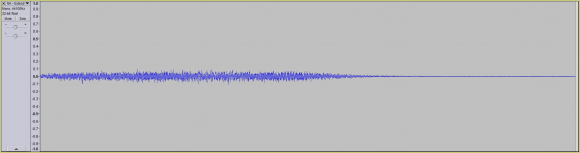...or just a portion (for example the first half of both signals):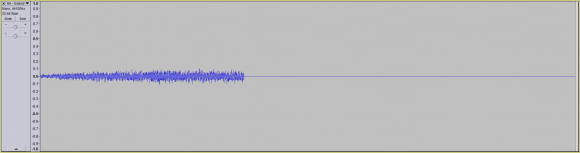...or the remaining halves of both signals: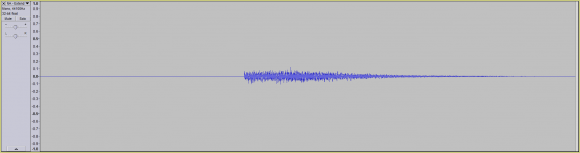Note: Notice how when comparing both signals, both signals are truncated at the same exact point in time (or not truncated at all).

So in all 3 groups of pairs, I would need this function to result in the same number (ex. 2.75).

However, if I was comparing both signals at different points (for example, comparing the 1st half of one signal with the 2nd half of the other signal):...the correlative would not hold.

So in other words, for this correlative value to hold, we'd need to be comparing both convolved signals at the same time range.

Apologies if this sounds a little confusing.

Thank you for all your help,
Nelson

[ - ]Thanks for the clarification. Because you are designing a function that accepts two vectors and outputs a value, it makes the most sense to me to implement it using an integral of some other function that accepts two vectors and outputs a vector, over all space/time. That function could be implemented using a ratio of the Fourier transforms of the two signals, such that any convolution in the time domain would be equivalent to a multiplication in the Fourier domain, and would therefor cancel out. This would require that neither impulse response, nor the signal be convolved, has any zeroes in the Fourier domain, otherwise you would need some way to handle the 1/0 case(s).

That being said, windowing the data could absolutely change the value of the output.  When you window the time domain signal, you are low pass filtering/changing the data in the fourier domain, and the math to cancel out the signal would no longer apply, even if you apply the same window to both input.

Unfortunately, I can't think of another function prototype that would work any better.

[ - ]Hello Dszabo,

Firstly I can't thank you enough for your solution.

I was trying out a few things myself, but wasn't able to figure anything out.

Quite frankly I didn't think it was possible (and still have my doubts).

That being said, to be absolutely certain that I adequately described the problem, allow me to offer a more detailed description of the problem (just in case I overlooked something).

If you feel that your solution still hold (or if it doesn't), if you could please let me know, I would greatly appreciate it.

Let's assume we start out with the following dry signal (DS):Using the following IR (A):...to convolve our dry signal (DS), results in the following wet signal (A(DS)):Whereas when using the following IR (B):...to convolve our dry signal (DS), results in the following wet signal (B(DS)):So to recap, we have:

1 dry signal (DS)
2 IR's (A and B).
2 wet / convolved signals (A(DS) and B(DS))

The first thing I'm trying to do is establish a mathematical correlation between both A(DS):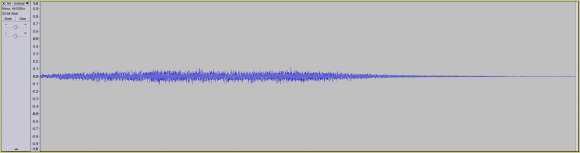...and B(DS):...such that the mathematical correlation between both A(DS) and B(DS) using your method is some number (ex. 2.75).

Furthermore, If we were to calculate the mathematical correlation between A(DS) and some other wet signal (ex. C(DS)), the correlative value would be different (ex. 5.79).

At this point I have no doubt that your method works.

Now what has me a little worried is the next part.

I need this mathematical correlation to stand (i.e. retain the same value of 2.75), whether I'm comparing the entirety of the time domain for both convolved signals:...or only some portion of the time domain:In other words in both situation, as long as we are comparing the same time domain for both A(DS) and B(DS), the same mathematical correlative value holds (ex. equating to a value of 2.75).

If you're certain that your mathematical solution still holds, the final thing I require from your solution is that if we're comparing different time domains for A(DS) and B(DS):...that the mathematical correlation not hold.

So in other words, for the mathematical correlation to hold, we must be comparing A(DS) and B(DS) across the same time domain (whether it be the entirety of both signals or just a portion of both signals).

Regardless of the length of the time domain, the correlative value must be the same (ex. 2.75).

The only time when the correlative may differ is when we're comparing one time domain for A(DS) (ex. 0 - 1 seconds) and a different time domain for B(DS) (ex. 1.25 - 2 seconds).

If you're certain that your solution still holds, I really can't thank you enough for all your help.

If on the other hand you don't feel your solution holds given the clarified / additional criteria, I'm still very thankful for all your help.

Nelson

[ - ]